The Parabola as a Parent Function MathBitsNotebook.com Terms of Use   Contact Person: Donna Roberts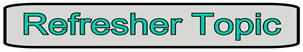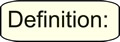A parent function is the simplest function of a family of functions. For the family of quadratic functions,  y = ax2 + bx + c, the simplest function of this form is y = x2.The "Parent" Graph: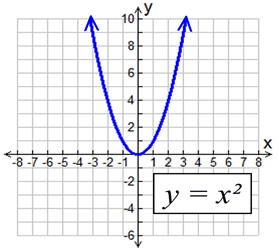The simplest parabola is y = x2, whose graph is shown at the right. The graph passes through the origin (0,0), and is contained in Quadrants I and II. This graph is known as the "Parent Function" for parabolas, or quadratic functions. All other parabolas, or quadratic functions, can be obtained from this graph by one or more transformations.The "Children" Graphs:The "parent" parabola can give birth to a myriad of other parabolic shapes through the process of transformations. Brush off your memories of transformations and let's take a quick look at what is possible. When graphing quadratic functions (parabolas), keep in mind that two forms of equations may be used:     y = ax2 + bx + c   or   y = a(x - h)2 + k
 Vertical Translation Horizontal Translation move the graph vertically - up or down y = x2+ k y = x2+ 4 moves the graph UP 4 units y = x2- 4 moves the graph DOWN 4 units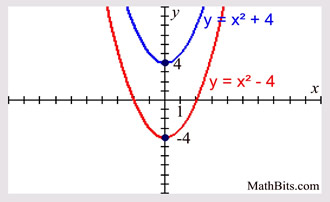move the graph horizontally - left or right y = (x - h)2 y = (x - 4)2 moves the graph RIGHT 4 units y = (x + 2)2 moves the graph LEFT 2 units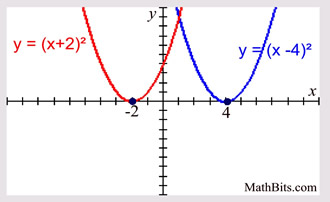Reflection in x-axis Reflection in y-axis flip the graph over the x-axis (negates the y-values of the coordinates) y = -(x2) y = x2 parent graph y = -(x2) parent reflected over x-axis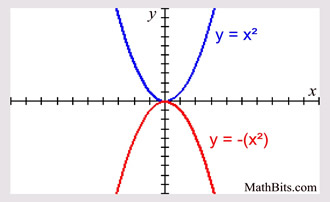flip the graph over the y-axis (negates the x-values of the coordinates) y = (-x)2 y = x2 parent graph y = (-x)2 parent reflected over y-axis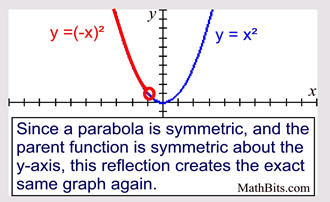Stretch or Compress Vertically Stretch or Compress Horizontally stretches away from the x-axis or compresses toward the x-axis y = a • x2 | a | > 1 is a stretch; 0 < | a | <1 is a compression y = x2 parent graph y = ¼(x)2 vertical compression y = 4(x)2 vertical stretch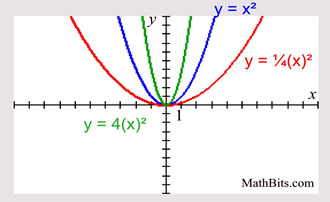stretches away from the y-axis or compresses toward the y-axis y = (a • x)2 | a | > 1 is a compression by factor of 1/a; 0 < | a | <1 is a stretch by factor of 1/a y = x2 parent graph y = (¼x)2 horizontal stretch y = (4x)2 horizontal compression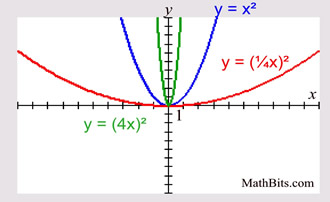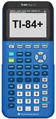For calculator help with graphing parabolas click here.For calculator help with examining transformations click here.